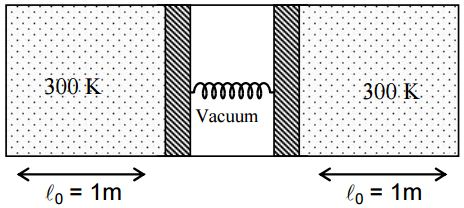# Find the Compression!Consider the diagram shown above where the two chambers separated by piston-spring arrangement contain equal amounts of same ideal gas. Initially when the temperatures of the gas in both the chambers are kept at $300 K$. The compression in the spring is $10 \text{ cm}$. The temperature of the left and the right chambers are now raised slowly to $400 K$ and $500 K$ respectively. The pistons are free to slide (i.e. no friction between piston and chamber). Final compression in the spring is found to be ($\frac{\alpha}{60}m$). Find $\alpha$.

Use $\sqrt{481} = 22$.

×